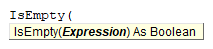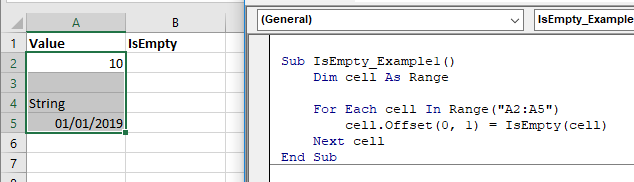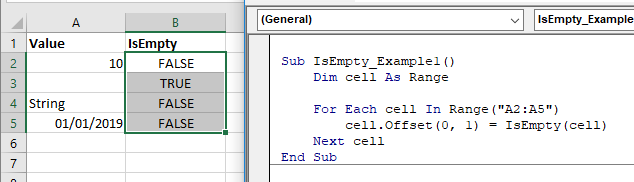# VBA IsEmpty Function

## IsEmpty Description

Used to check for blank cells or uninitialized variables.

## Simple IsEmpty Examples

``````Sub IsEmpty_Example()
Dim a As Variant
MsgBox IsEmpty(a)
End Sub``````

This will return True.

``````Sub IsEmpty_Example()
Dim a As Variant
a = 10
MsgBox IsEmpty(a)
End Sub``````

This will return False.

## IsEmpty Syntax

In the VBA Editor, you can type  “IsEmpty(” to see the syntax for the IsEmpty Function:The IsEmpty function contains an argument:

Expression: An expression that will be evaluated.

## Examples of Excel VBA IsEmpty Function

``````Dim a As Variant
a = Null
MsgBox IsEmpty(a)``````

Result: False

``````Dim a As Variant
a = Empty
MsgBox IsEmpty(a)``````

Result: True

IsEmpty function can be used to check blank cells.``````Sub IsEmpty_Example1()
Dim cell As Range

For Each cell In Range("A2:A5")
cell.Offset(0, 1) = IsEmpty(cell)
Next cell
End Sub``````

The result is as following.Stop searching for VBA code online. Learn more about AutoMacro - A VBA Code Builder that allows beginners to code procedures from scratch with minimal coding knowledge and with many time-saving features for all users!